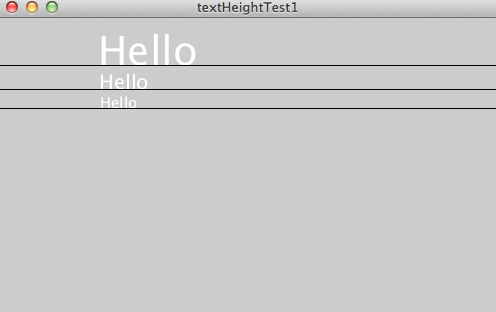#### Howdy, Stranger!

We are about to switch to a new forum software. Until then we have removed the registration on this forum.

# How do I do this using arrays and a for loop?

``````size(500, 300);

textAlign(LEFT);

textSize(40);
float h1 = textAscent() + textDescent();
text("Hello", 100, h1);
line(0, h1, width, h1);

textSize(20);
float h2 = textAscent() + textDescent();
text("Hello", 101, h2+h1);
line(0, h2+h1, width, h2+h1);

textSize(15);
float h3 = textAscent() + textDescent();
text("Hello", 102, h3+h2+h1);
line(0, h3+h2+h1, width, h3+h2+h1);
``````

So that the y position of the "current" line is the sum of the heights of the previous lines and the height of its own. Looks like this at the moment:Tagged:

• edited October 2014 Answer ✓
``````// forum.processing.org/two/discussion/7793/
// how-do-i-do-this-using-arrays-and-a-for-loop

size(500, 300, JAVA2D);
noLoop();
smooth(4);
textAlign(LEFT, BASELINE);

stroke(-1);
strokeWeight(1.5);

fill(#FFFF00);
background(0);

for (int heightSum = 0, x = 100, i = 40; i >= 10; ++x, i -= 5) {
textSize(i);
heightSum += textAscent() + textDescent();
text("Hello", x, heightSum);
line(0, heightSum, width, heightSum);
}
``````
• Thank you very much.